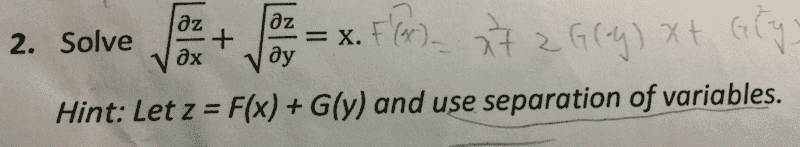# Partial Differential Equation with square roots

Johnson Chou
<Moderator's note: Moved from a technical forum and thus no template.>

Hi everyone,
I have encountered a partial differential equation with square roots which I don't have a clue in solving it. After letting z=F(x)+G(y), I can't really figure out the next step. I tried squaring both sides but the square root still exists. Any help would be appreciated, thank you!#### Attachments

Last edited by a moderator:

Homework Helper
Hello Johnson,Please post in a homework forum and use the template. See Guidelines . We need an attempt at solution (posted) to be allowed to help out.

In the mean time:
How would you solve ##\sqrt{\partial z\over \partial x} = x ## ?

Johnson Chou
\begin{aligned}\dfrac {\partial z}{\partial x}=x^{2}\\ \partial z=x^{2}\partial x\\ z=\dfrac {x^{3}}{3}+c\end{aligned}
My attempt to my problem are as follow:
I try to reproduce the equation to a 1st order differential equation,
Let z(x,y)= F(x)+G(y)
Therefore the equation becomes (F'(x))^1/2+(G'(y))^1/2=x
Taking square on both sides still doesn't eliminate the the square root and squaring it one more time involves F'(x)^2.

Last edited by a moderator:
Homework Helper
Good. Notice anything remarkable in relation to your problem ?

Johnson Chou
Sorry I don't quite understand the relation, but my attempt on continuing the question with your hint:
\begin{aligned}\sqrt {\dfrac {\partial z}{\partial x}}+\sqrt {\dfrac {\partial z}{\partial y}}=x\\
\sqrt {\dfrac {\partial z}{\partial x}}=x\ & \sqrt {\dfrac {\partial z}{\partial y}}=0\\
\therefore \dfrac {\partial z}{\partial x}=x^{2},\dfrac {\partial z}{\partial y}=0\\
z=\dfrac {x^{3}}{3}+G\left( y\right) =\dfrac {x^{3}}{3}+C\end{aligned}

Last edited by a moderator:
That's what I meant: the solution to my problem is also a solution for your problem•That's what I meant: the solution to my problem is also a solution for your problem